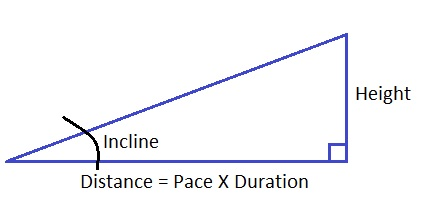# EXERCISE

## Calorie Calculation

### You will burn calories without using an incline. Adding an incline, adds work and will burn more.

• Pace
• Duration
• InclineWEIGHT:     Pounds

AVG SPEED:   MPH

DURATION:    Minutes

ANGLE:     Degrees

ESTIMATED CALORIES:   ________

Added calories burned (energy) = Distance elevated X Weight
Distance elevated = Height
Incline Energy = Height * Weight

Tangent = Opposite / Adjacent
---------------------------------------
Tan(Incline) = Height / Distance
Height / Distance = Tan(Incline)
Height = Tan(Incline) * Distance
Height = Distance * Tan(Incline)
Height = Pace * Duration * Tan(Incline)

Incline Energy = Height * Weight
Incline Energy = Pace * Duration * Tan(Incline) * Weight

Estimated Incline Calories = Energy       Estimated No Incline Calories = Speed * Duration

YMCA
15 Witts End
Hamburg, New Jersey 07419
866-930-8546
William F. Baur